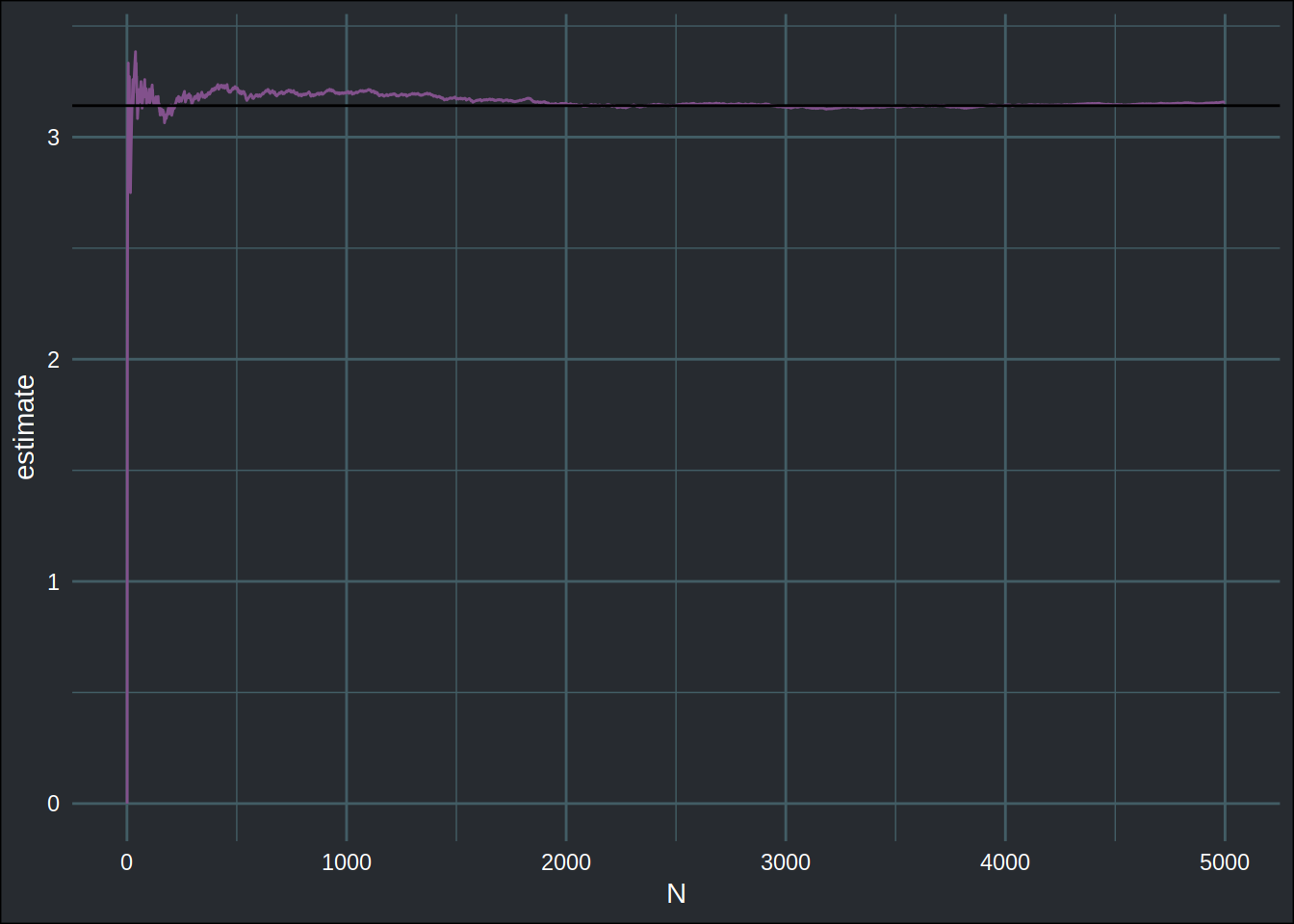Econometrics and Free Software by Bruno Rodrigues.
Check out my package that adds logging to R functions, {chronicler}.
Or read my free ebooks, to learn some R and build reproducible analytical pipelines..
You can also watch my youtube channel or find the slides to the talks I've given here.
Buy me a coffee, my kids don't let me sleep.


# Using the tidyverse for more than data manipulation: estimating pi with Monte Carlo methods

R

This blog post is an excerpt of my ebook Modern R with the tidyverse that you can read for free here. This is taken from Chapter 5, which presents the {tidyverse} packages and how to use them to compute descriptive statistics and manipulate data. In the text below, I show how you can use the {tidyverse} functions and principles for the estimation of $$\pi$$ using Monte Carlo simulation.

## Going beyond descriptive statistics and data manipulation

The {tidyverse} collection of packages can do much more than simply data manipulation and descriptive statisics. You can use the principles we have covered and the functions you now know to do much more. For instance, you can use a few {tidyverse} functions to do Monte Carlo simulations, for example to estimate $$\pi$$.

Draw the unit circle inside the unit square, the ratio of the area of the circle to the area of the square will be $$\pi/4$$. Then shot K arrows at the square; roughly $$K*\pi/4$$ should have fallen inside the circle. So if now you shoot N arrows at the square, and M fall inside the circle, you have the following relationship $$M = N*\pi/4$$. You can thus compute $$\pi$$ like so: $$\pi = 4*M/N$$.

The more arrows N you throw at the square, the better approximation of $$\pi$$ you’ll have. Let’s try to do this with a tidy Monte Carlo simulation. First, let’s randomly pick some points inside the unit square:

library(tidyverse)
library(brotools)
n <- 5000

set.seed(2019)
points <- tibble("x" = runif(n), "y" = runif(n))

Now, to know if a point is inside the unit circle, we need to check wether $$x^2 + y^2 < 1$$. Let’s add a new column to the points tibble, called inside equal to 1 if the point is inside the unit circle and 0 if not:

points <- points %>%
mutate(inside = map2_dbl(.x = x, .y = y, ~ifelse(.x**2 + .y**2 < 1, 1, 0))) %>%
rowid_to_column("N")

Let’s take a look at points:

points
## # A tibble: 5,000 x 4
##        N       x      y inside
##    <int>   <dbl>  <dbl>  <dbl>
##  1     1 0.770   0.984       0
##  2     2 0.713   0.0107      1
##  3     3 0.303   0.133       1
##  4     4 0.618   0.0378      1
##  5     5 0.0505  0.677       1
##  6     6 0.0432  0.0846      1
##  7     7 0.820   0.727       0
##  8     8 0.00961 0.0758      1
##  9     9 0.102   0.373       1
## 10    10 0.609   0.676       1
## # … with 4,990 more rows

The rowid_to_column() function, from the {tibble} package, adds a new column to the data frame with an id, going from 1 to the number of rows in the data frame. Now, I can compute the estimation of $$\pi$$ at each row, by computing the cumulative sum of the 1’s in the inside column and dividing that by the current value of N column:

points <- points %>%
mutate(estimate = 4*cumsum(inside)/N)

cumsum(inside) is the M from the formula. Now, we can finish by plotting the result:

ggplot(points) +
geom_line(aes(y = estimate, x = N), colour = "#82518c") +
geom_hline(yintercept = pi) +
theme_blog()In Chapter 6, we are going to learn all about {ggplot2}.

As the number of tries grows, the estimation of $$\pi$$ gets better.

Using a data frame as a structure to hold our simulated points and the results makes it very easy to avoid loops, and thus write code that is more concise and easier to follow. If you studied a quantitative field in u8niversity, you might have done a similar exercise at the time, very likely by defining a matrix to hold your points, and an empty vector to hold whether a particular point was inside the unit circle. Then you wrote a loop to compute whether a point was inside the unit circle, save this result in the before-defined empty vector and then compute the estimation of $$\pi$$. Again, I take this opportunity here to stress that there is nothing wrong with this approach per se, but R, with the {tidyverse} is better suited for a workflow where lists or data frames are the central objects and where the analyst operates over them with functional programming techniques.

Hope you enjoyed! If you found this blog post useful, you might want to follow me on twitter for blog post updates and buy me an espresso or paypal.me.